# Quiz 18: Asymmetric Information

a) If the contingency fee is 1/3 rd , the surplus to the lawyer is given by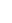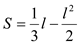The first order condition for the above function is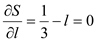Hence, the surplus maximizing effort for the lawyer is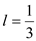The lawyer's surplus then isThe plaintiff's surplus then is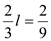b) If the contingency fee is c , the surplus to the lawyer is given by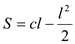The first order condition for the above function is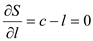Hence, the surplus maximizing effort for the lawyer is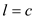The lawyer's surplus then is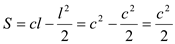The plaintiff's surplus then is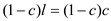c) The plaintiff's surplus is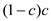The first order condition for the above function is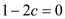Hence, the surplus maximizing contingency fee for the plaintiff is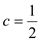The plaintiff's surplus then is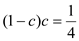If contingency fees is, surplus maximizing effort for the lawyer is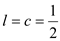The lawyer's surplus then is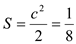d) If the plaintiff can sell the case to her lawyer she can extract the whole surplus of the lawyer and increase her surplus. This can be done by setting a 100% contingency fee. The lawyer will then choose l = 1 and earn a surplus ½. The plaintiff can then sell the case for ½ and extract his surplus. As this scheme does not leave the lawyer with any surplus it is outlawed in many countries.
If instead of two tariffs, the coffee shop charges price p per ounce of coffee, then the the utility function of a consumer is given by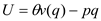The first order condition for the above yields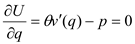So, the inverse demand function of consumer is given by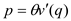As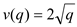, we get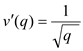Putting this in the inverse demand function, we get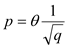So,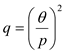The expected profit of the coffee shop is given by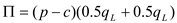Substituting the demand function for the two types of demanders, we get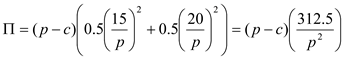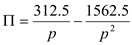The first order condition for this is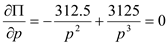Re arranging the above we get,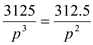Solving for p, we get,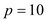The expected profit of the shop is given by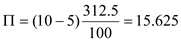If instead the firm sets non-linear price, the expected profit is found to be 50. Hence, non-linear price is a better strategy.
a) We know that to maximize the monopolists' expected profit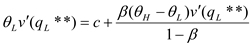As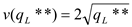, we get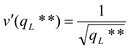Substituting the values, we get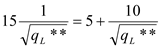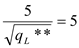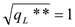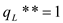The tariff for the low type is given by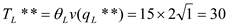The optimal quantity and tariff for high demander remains unchanged at 16 and 160 respectively. Only the quantity and tariff of the low type is affected, that of the high type remains unaffected. This shows that there is "no distortion at the top".